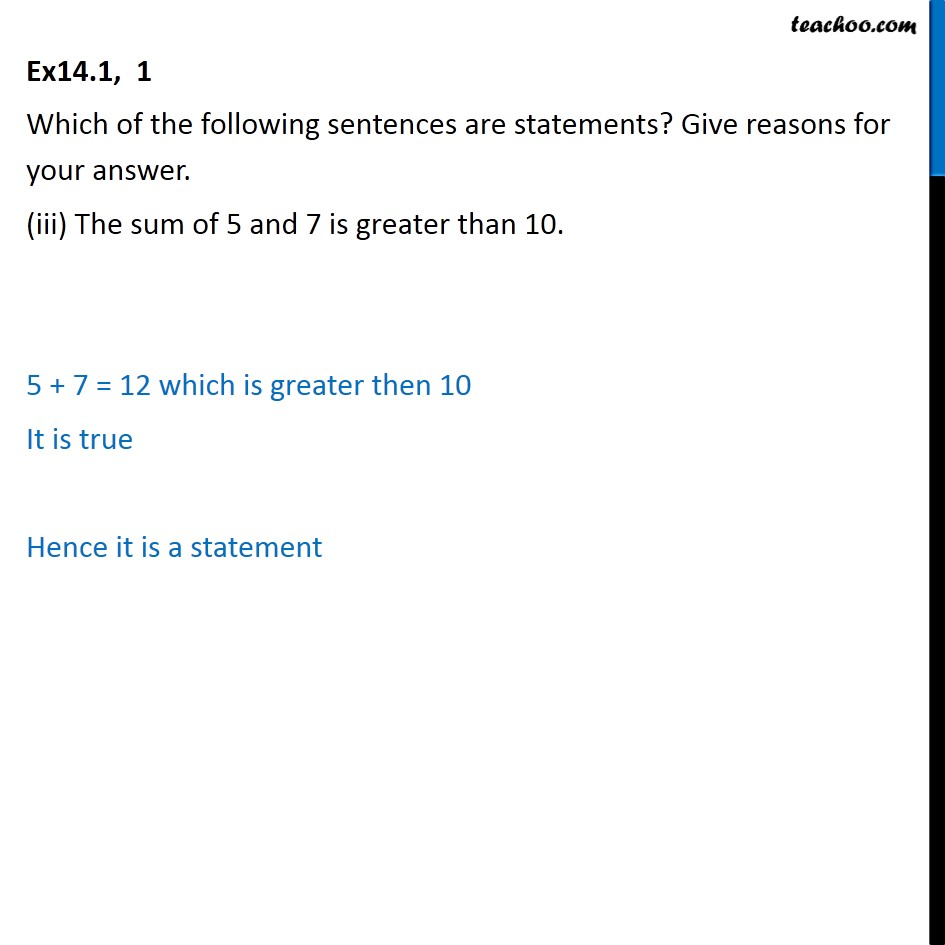1. Chapter 14 Class 11 Mathematical Reasoning (Deleted)
2. Serial order wise
3. Ex 14.1

Transcript

Ex14.1, 1 Which of the following sentences are statements? Give reasons for your answer. (iii) The sum of 5 and 7 is greater than 10. 5 + 7 = 12 which is greater then 10 It is true Hence it is a statement